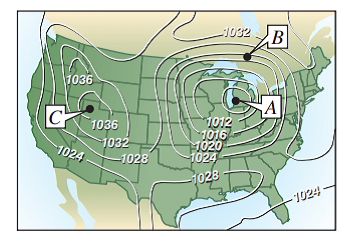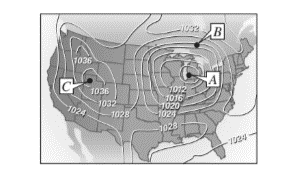Chapter 7.3, Problem 51E### Calculus: An Applied Approach (Min...

10th Edition
Ron Larson
ISBN: 9781305860919

#### Solutions

Chapter
Section### Calculus: An Applied Approach (Min...

10th Edition
Ron Larson
ISBN: 9781305860919
Textbook Problem
1 views

# Meteorology Meteorologists measure the atmospheric- pressure in millibars. From these observations they create weather maps on which the curves of equal atmospheric pressure (isobars) are drawn (see figure). On the map, the closer the isobars, the higher the wind speed. Match points A, B, and C with (a) highest pressure, (b) lowest pressure, and (c) highest wind speed.NASA

To determine

The correct match for point A, B and C with (a) highest pressure (b) lowest pressure, and (c) highest wind speed by using curve of equal atmospheric pressure (isobars) are given below,Explanation

Given Information:

The isobars curve of equal atmospheric pressure (isobars) are given below,

The closer the isobars, highest the pressure,

Consider the map of isobars curve of equal atmospheric pressure (isobars),

### Still sussing out bartleby?

Check out a sample textbook solution.

See a sample solution

#### The Solution to Your Study Problems

Bartleby provides explanations to thousands of textbook problems written by our experts, many with advanced degrees!

Get Started

#### Differentiate. g() = e(tan )

Single Variable Calculus: Early Transcendentals, Volume I

#### In Exercises 3540, rationalize the numerator of each expression. 37. 133

Applied Calculus for the Managerial, Life, and Social Sciences: A Brief Approach

#### In problems 37-44, perform the indicated operations and simplify. 41.

Mathematical Applications for the Management, Life, and Social Sciences

#### Find the unit tangent vector for at t = –1.

Study Guide for Stewart's Multivariable Calculus, 8th